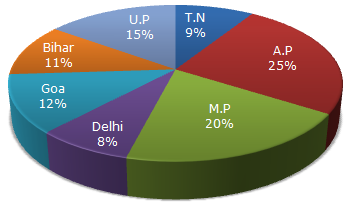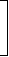# Data Interpretation - Pie Charts - Discussion

Study the following graph and the table and answer the questions given below.

Data of different states regarding population of states in the year 1998Total population of the given States = 3276000.

 States Sex and Literacy wise Population Ratio Sex Literacy M F Literate Illiterate A.P 5 3 2 7 M.P 3 1 1 4 Delhi 2 3 2 1 Goa 3 5 3 2 Bihar 3 4 4 1 U.P. 3 2 7 2 T.N. 3 4 9 4

1.

What will be the percentage of total number of males in U.P., M.P. and Goa together to the total population of all the given states?

 [A]. 25% [B]. 27.5% [C]. 28.5% [D]. 31.5%

Explanation:

 Number of males in U.P =3 of (15% of N)= 3 x 15 x N   = 9 x N . 5 5 100 100

where N = 3276000.

 Number of males in M.P =3 of (20% of N)= 3 x 20 x N   = 15 x N . 4 4 100 100

 Number of males in Goa =3 of (12% of N)= 3 x 12 x N   = 4.5 x N . 8 8 100 100Total number of males in these three states
 = (9 + 15 + 4.5) x N 100
 =28.5 x N. 100Required Percentage =28.5 x N100
x 100% = 28.5%.
N

 Sathish said: (Jun 2, 2011) Can't get how 3/5 comes in step 1 ?

 Sundar said: (Jun 2, 2011) How 3/5 comes at first step? Ans: Ratio of males and females in UP = 3 : 2 Number of Males = 3/(3+2) = 3/5 of 15% of N.

 Shailesh said: (May 2, 2012) Can any one tell me that how did come 15%, 20% and 12%?

 Rajapriya said: (Mar 11, 2013) Shailesh. See the pie chart. Its show the percentage. UP - 15%. MP - 20%. Goa - 12%. I Hope that you Got My Point.

 Rani said: (Apr 8, 2013) Directly we can take the male percentages to total. i.e. U.P. Male = 3% of 15% 3276000. Like this we can take M.P and GOA then we add.

 Sai said: (Apr 17, 2014) Can anyone tell me how did come 15%? I cannot understand this problem?

 Raquel said: (Jul 5, 2015) Does any one have a simpler solution to this problem? I don't understand the working out.

 Rithish said: (Feb 25, 2016) U.P (Male, Female ratio) = 3 : 2 = 3/(3 + 2) = 3/5. M.P = 3:1 = 3/4. Goa = 3:5 = 3/8. We know that 3276000 value remains same for all states. So, take only the percentages and assume 3276000 as 'N'. U.P = Population percentage = 15%. M.P = 20%. Goa = 12%. No of men in U.P = 3/5 (15/100) N = (9/100) N. M.P = 3/4 (20/100) N = (15/100) N. Goa = 3/8 (12/100) N = (4.5/100) N. Adding these three, we get (28.5/100) N. Required % = {(28.5/100)N/N } * 100 = 28.5%.

 Lisa said: (Mar 22, 2016) How do you get the answers of 9, 14 and 4.5?

 Akhilesh said: (Sep 2, 2016) What is the average score of this test? Please tell me the derivation part.

 Anonymous said: (Nov 16, 2017) In the question they didn't mention that the men and female are in the ratio.

 Riri said: (Apr 5, 2018) Here They have taken the whole ratio of the population of M +F.

 Jimmy said: (Jul 23, 2018) How come a number of people in decimals, Like 4.5? Please, can anyone explain me?

 Ugyen said: (Jul 25, 2018) Why we have to add 3+2 in the first step?